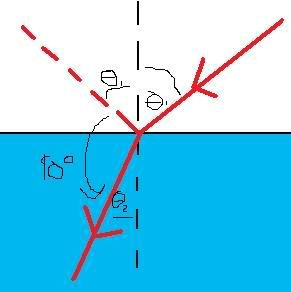October 19, 2019, 10:53:09 pmAuthorTopic: Relative Refractive Index question!  (Read 621 times) Tweet Share

0 Members and 1 Guest are viewing this topic.

squance

• GuestRelative Refractive Index question!
« on: January 30, 2008, 11:02:42 am »
0
At what angle of incidence should a beam of light strike the surface of a still pond if the angle between the reflected ray and the refracted ray is to be 90 degrees? (n=1.33 for water)

I have no idea how to solve this or what this is about (this is for my sis whos doing yr 11 physics)

cara.mel

• GuestRe: Relative Refractive Index question!
« Reply #1 on: January 30, 2008, 11:43:11 am »
0
EDIT: SHINY PICTURE NOW:After this, I can't be bothered learning latex, pretend x1 and x2 are theta 1 and 2

From this, we can see x1+x2 = 90º => x2=90-x1
Using our mate snell's law as per normal, 1 sin x1 = 1.33 sin x2
sin x1 = 1.33 sin (90-x1)
sin x1 = 1.33 cos x1
tan x1 = 1.33
x1 = 53º

Edit: Today when studying for my exam at 2:30 I learnt of Brewsters Law, which says if the reflected and refracted rays are perpendicular, then theta = arctan(n_2/n_1)

Which is essentially what I derived by doing arctan(1.33/1)« Last Edit: June 17, 2008, 08:59:00 am by caramel »

squance

• GuestRe: Relative Refractive Index question!
« Reply #2 on: January 30, 2008, 11:52:40 am »
0
Thanks!! My sis will now prob be able to draw it herself and understand it!